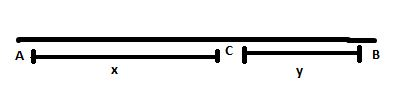# Why Is The Speed, In General, Greater Than The Magnitude Of The Velocity?

From the definitions, we know that

$$\begin{array}{l}Speed = \frac{Distance\;travelled}{Time\;taken}\end{array}$$

And

$$\begin{array}{l}Velocity = \frac{Displacement\;of\;body}{Time\;taken}\end{array}$$

Consider the figure given below:From the figure, speed and velocity can be given as:

$$\begin{array}{l}Speed = \frac{AB+BC}{t}\end{array}$$

And

$$\begin{array}{l}Velocity = \frac{AC}{t}\end{array}$$

Substituting the values, we get

$$\begin{array}{l}Speed = \frac{x+2y}{t}\end{array}$$

And

$$\begin{array}{l}Velocity = \frac{x}{t}\end{array}$$

Therefore, speed is greater than speed.(4)(1)